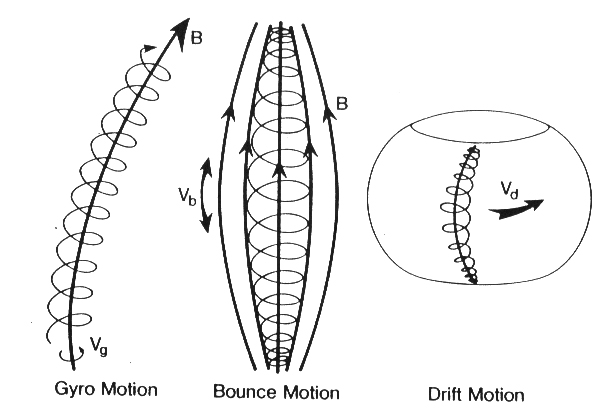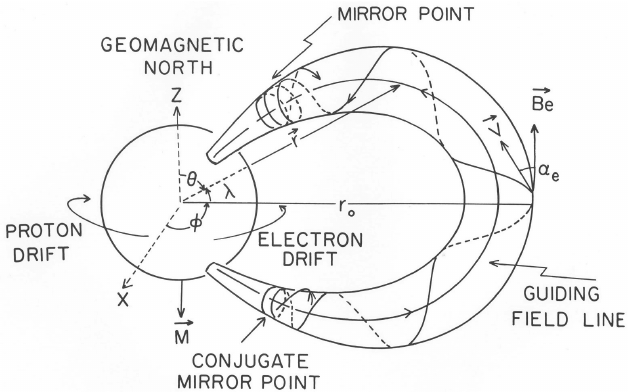When charged particles move within  magnetic field, they may be trapped along the field lines in three superimposed motions: $$spiral$$, $$bounce$$ and $$drift$$, The understanding of the trapping mechanism is extremely important for the interpretation of the space environment wich affects most of the cosmic rays measurements of satellite detectors in the magnetosphere. The trapping principles are best explained with $$adiabatic \: invariants$$, which are approximate constants of the motionof charged particles in the geomagnetic field, varying extremely slowly with time (adiabatic).The first kind of motion that a charged particle has in a magnetic field is a gyration around the field lines. If the magnetic field is $$\overrightarrow{B}$$ the frequency of gyration is $$\omega_{B}=\frac{eB}{m}$$ and the gyroradius is $$r_{B}=\frac{mv_{\perp}}{Be}$$ where $$v_{\perp}$$ is the component of the velocity perpendicular to the magnetic field and $$v_{\Vert}$$ is parallel. In this case the particle trajectory is a spiral. If the only force acting on the particle is the magnetic one, no work is done on the particle and the magnetic flux $$\phi_{m}$$ is constant, so:

\begin{equation}\label{eq:fluxmag}
\phi_{m}=B \pi r^{2}_{B}=\frac{2\pi m E^{\perp}_{k}}{e^{2}B}
\end{equation}

where $$E^{\perp}_{k}$$ is the kinetic energy associated with the transverse motion, the velocity component  $$v_{\perp}$$, and so is $$\frac{mv^{2}_{\perp}}{2}$$. From  the invariance of $$\phi_{m}$$, eq. \ref{eq:fluxmag}, we have $$\frac{E^{\perp}_{k}}{B}=constant$$ so the magnetic moment of the current loop $$\mu$$ given by:

\begin{equation}\label{eq:mu}
\mu = i\times A = \frac{ev_{\perp}}{2 \pi r_{B}}\pi r^{2}_{B} = \frac{E^{\perp}_{k}}{B}
\end{equation}

is constant too, and $$\mu$$ is called $$first \: adiabatic \: invariant$$. This invariant is related to the bounce motion (motion parallel to the field lines). The invariance of $$\frac{E^{\perp}_{k}}{B}$$ also applies if the particle moves into a region of different $$B$$, either by following a field line or by drifting across field lines. We can now obtain the most frequently used equation in radiation belts physics. Calling $$pitch \: angle$$ $$\alpha$$ the angle bewtween perpendicular and parallel velocity component (so $$\tan \alpha = \frac{v_{\perp}}{v_{\Vert}}$$)

\begin{equation}\label{eq:alpha0}
\frac{E_{k}\sin ^{2} \alpha}{B} = constant
\end{equation}

Because the ratio $$\frac{E^{\perp}_{k}}{B}$$ is constant, when a particle moves toward the Earth magnetic pole, $$v_{\perp}$$ and $$\sin^{2}\alpha$$ must increase (the magnetic field $$B$$ increases), eventually reaching the value $$\alpha=90^{o}$$. In this point the particle motion is reversed and this is called the $$mirror \:point$$ (that correspond to a value $$B_{m}$$ or mirror field). This mechanisn approximate the $$magnetic \: bottle$$ mechanism in a non-uniform field. The total energy of the particle is not changing, because no acceleration mechanisms are at work (in the absence of electric field), so $$v$$ is constant and calling $$B_{0}$$ and $$\alpha_{0}$$ the values of the magnetic field and the pitch angle at the geomagnetic equator, we can write the relation

\begin{equation}\label{eq:alpha}
\frac{B_{0}}{B_{m}}=\frac{\sin^{2} \alpha_{0} }{\sin^{2} 90^{o} }=\sin^{2} \alpha_{0}
\end{equation}

If particles encounter the atmosphere before they bounce back they will be lost by absorption (interaction) with it, this happens for all particles that in any location $$X$$ have a picth angle $$\alpha _{LC}$$ that is $$\alpha < \alpha_{LC}$$, where

\begin{equation}\label{eq:loss}
\sin^{2} \alpha_{LC}= \frac{B_{X}}{B_{a}}
\end{equation}

where $$B_{X}$$ is the intensity in $$X$$ and $$B_{a}$$ is the intensity at the intersection of the field line with the atmosphere (typically $$\simeq 100 \: km$$.

There are two more adiabatic invariants, the first (namely the $$second \: adiabatic \: invariant$$) one is related to the motion along field lines, between the mirror points, the so called bouncing motion. The parallel component of particle momentum can be written as

\begin{equation}\label{eq:parall}
p_{||} = p \cos \alpha = p\sqrt{1-\frac{B}{B_{m}}}
\end{equation}

A particle can move along a field line until the magnetic field becomes equal to the maximum field. It will then turn and move back towards weaker regions. the pitch angle is minumum at the magnetic equator during each bouncing, and becomes $$90^{o}$$ at mirror points in the northern and southern hemispheres as the particle penetrates into stronger magnetic field regions. If the field variations are small during a particle bounce  period,  the second adiabatic invariant is expressed as:

\begin{equation}\label{eq:second}
J_{2} = p \int^{A}_{A'} \sqrt{1-\frac{B}{B_{m}} dl_{b}}
\end{equation}

or better $$I_{}=\frac{J_{2}}{2p} \simeq constant$$. This is an adiabatically conserved quantity along the bouncing motion, and for a given particle's momentum is completely defined by the structure of the field. It is a property of the field configurations and also of the mirror points of the particle and defines a surface, or $$shell$$, on which the particle remains as it drifts around the Earth. It holds provided the field does not change appreciably during one bounce period. Taken together, the first and the second invariants define the locus of the mirror points of a bouncing and drifting trapped particle.The $$third \: adiabatic \: invariant$$ is related to a slowly changing magnetic field, where first and second adiabatic invariants (usually conserved in a static magnetic field) are still conserved, the particle momentum may change, but the magnetic flux in a drift path is conserved. It is related to the effect called the $$curvature-gradient \: drift$$. This effect is due to both the gradient of the field approaching the Earth and the curvature of the field lines that both produce a drift motion in the plane perpendicular to the dipole axis. The first effect is that the particle finds itself in a slightly weaker field when on the part of the gyration farthest from the Earth, the radius of the gyration therefore changes and this introduces a lateral shift to the orbit. The curvature of the field line produces a centrifugal force to which the particle responds by drifting sideways. So electrons are driven eastward and protons westwards. In a dipole field this effect would move the particles around at the same distance from the Earth (or the dipole center) and would serve merely to distribute the particles at all longitudes. However in the actual field, that is not exactly dipolar, the drift paths are not so obvious.

The expression of the $$third \: adiabatic \: invariant$$ is:

\begin{equation}\label{eq:third}
J_{3} = \int (q \overrightarrow{A}+ \overrightarrow{p})d\overrightarrow{l}_{d} \simeq q\phi \simeq constant
\end{equation}

where $$\phi$$ is the magnetic flux enclosed in the drift path. The invariance is violated if changes occur in a time $$t< \tau_{d}$$, where $$\tau_{d}$$ is the drift period.
Magnetic trapping occurs if the respective periods have a strict jerarchy, so $$\tau_{g} < \tau_{b}< \tau_{d}$$, where $$\tau_{g}$$ is gyration period and $$\tau_{b}$$ is the bouncing period.### McIlwain's L-parameter

An important development from the second invariantis the introduction of $$McIlwain's \: L \: parameter$$. It is a dimensionless parameter, taking the Earth radius as the unit:

\begin{equation}\label{eq:mcilwain}
L =  \frac{r_{0}}{r_{E}}
\end{equation}

Where $$r_{E}=6371.2 \: Km$$, In a perfect dipole it is recognized the convenience of a parameter $$r_{0}$$, the distance from the dipole center to the equatorial crossing or minimum $$B$$ value of a field line. During the particle bounce and drift motion, the particle remains on field lines having the same $$r_{0}$$. in the distorted geomagnetic field, position on the same fiel line will have very nearly the same value of $$L$$. This a particle which bounces and drifts around the Earth will be very near to an $$L$$ constant shell and can be assumed to follow an $$L$$ constant path. By analogy with a doipole field, an $$invariant \: latitude$$ may be defined in terms of $$L$$:

\begin{equation}\label{eq:tetamc}
\theta =  \cos^{-1}(1/L) ^{1/2}
\end{equation}

$$L$$ is commonly used to labe field-lines even at high latitude where $$L$$ strictly has no meaning because particles cannot be trapped on field lines that are not closed.